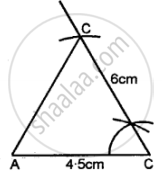# Construct Traingle Abc, When : Ac = 4.5 Cm, Bc = 6 Cm and ∠C = 60°. - Mathematics

Diagram
Sum

Construct traingle ABC, when : AC = 4.5 cm, BC = 6 cm and ∠C = 60°.

#### Solution

Steps of Construction:

(1) Draw a line AC = 4.5 cm.\

(2) With the help of compasses, construct ∠ACB = 60°.

(3) With C as centre, draw an arc of 6 cm radius, which cuts CB at C.

(4) Join B and A.

Concept: Classification of Triangles (On the Basis of Sides, and of Angles)
Is there an error in this question or solution?

#### APPEARS IN

Selina Class 6 Mathematics
Chapter 26 Triangles (Including Types, Properties and Constructions)
Exercise 26 (B) | Q 6